August 14, 2017

# Notes On Screen Space HIZ Tracing

The following is a small gathering of notes and findings that we made throughout the implementation of hiz tracing in screen space for ssr in Stingray. I recently heard a few claims regarding hiz tracing which motivated me to share some notes on the topic. Note that I also wrote about how we reproject reflections in a previous entry which might be of interest. Also note that I've included all the code at the bottom of the blog.

## SSR

The original implementation of our hiz tracing method was basically a straight port of the "Hi-Z Screen-Space Tracing" described in GPU-Pro 5 by Yasin Uludag. The very first results we got looked something like this:

Original scene: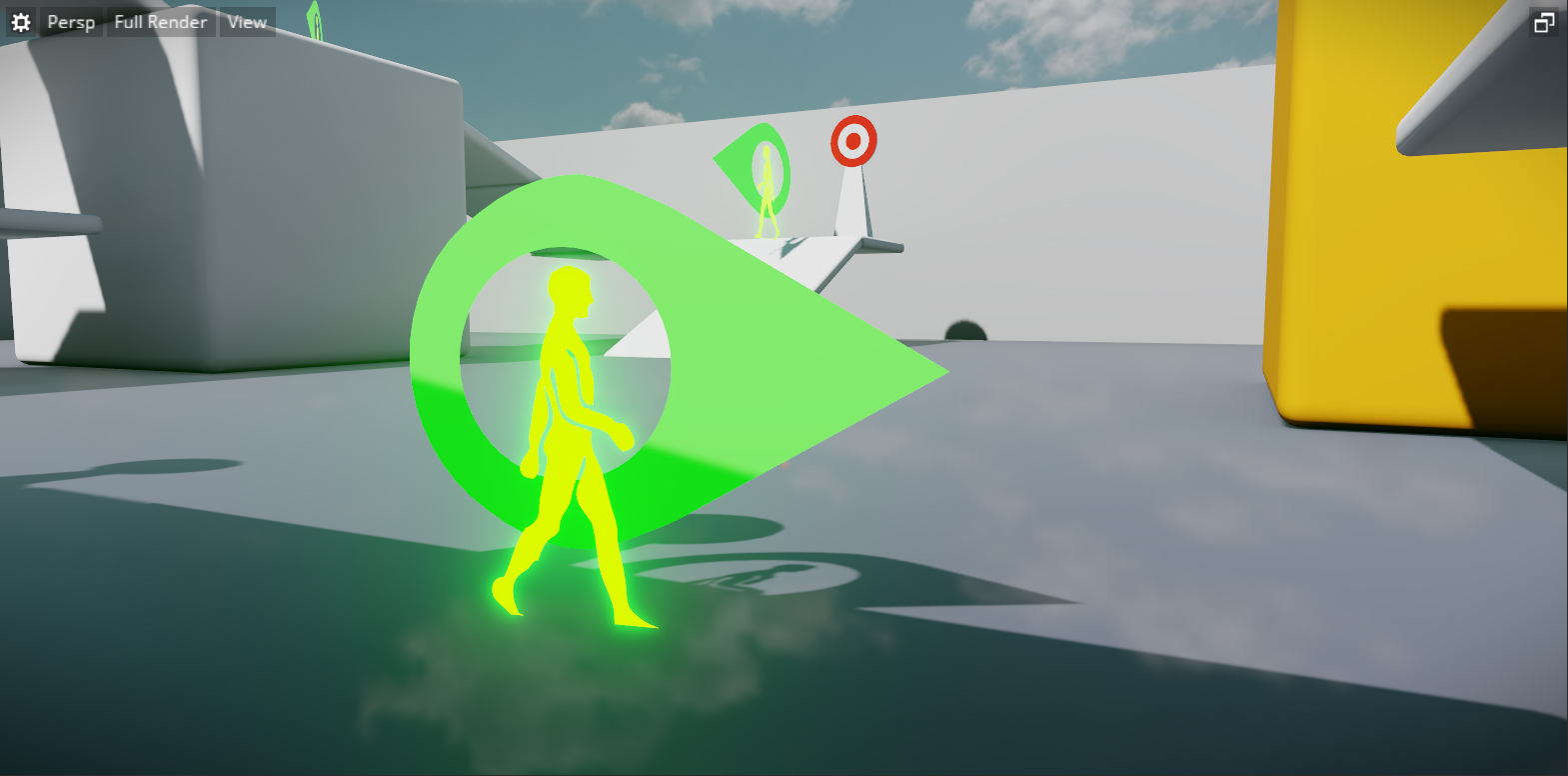Traced ssr using hiz tracing:## Artifacts

Some weird horizontal stripes were reported when ssr was enabled in the Stingray editor. They only revealed themselves for certain resolution (they would appear and disappear as the viewport got resized). I started writing some tracing visualization views to help me track each hiz trace event: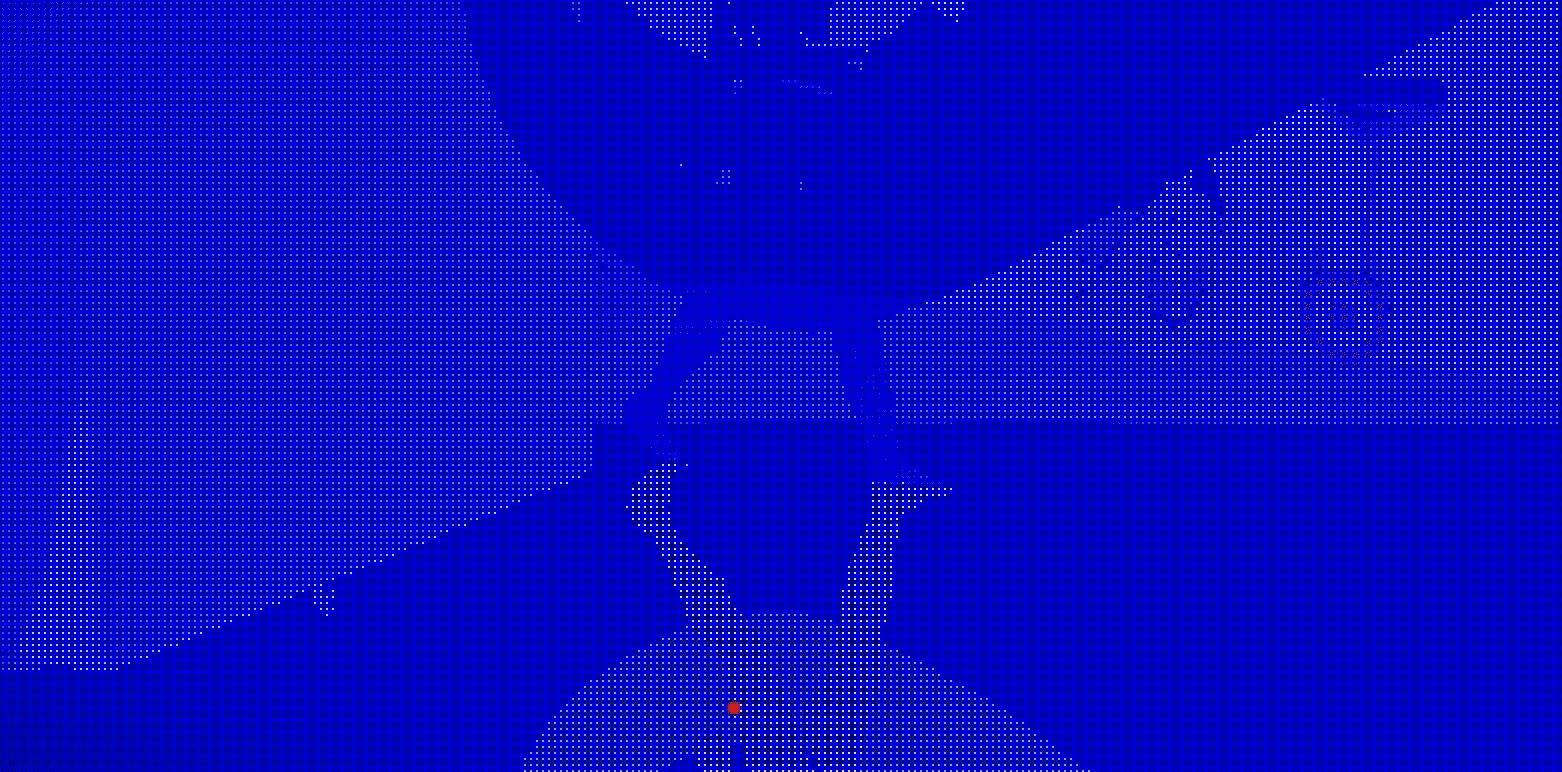Using these kinds of debug views I was able to see that for some resolution, the starting position of a ray when traced at half-res happened to be exactly at the edge of a hiz cell. Since tracing the hiz structure relies on intersecting the current position of a ray with the boundary of cell it lies in, it means that we need to do a ray/plane intersection. As the numerator of (planes - pos.xy)/dir.xy got closer and closer to zero the solutions for the intersection started to loose precision until it completely fell apart.

To tackle this problem we snap the origin of each traced rays to the center of a hiz cell:

``````float2 cell_count_at_start = cell_count(HIZ_START_LEVEL);
float2 aligned_uv = floor(input.uv * cell_count_at_start)/cell_count_at_start + 0.25/cell_count_at_start;``````

Rays traced with and without snapping to starting pos of the hiz cell center: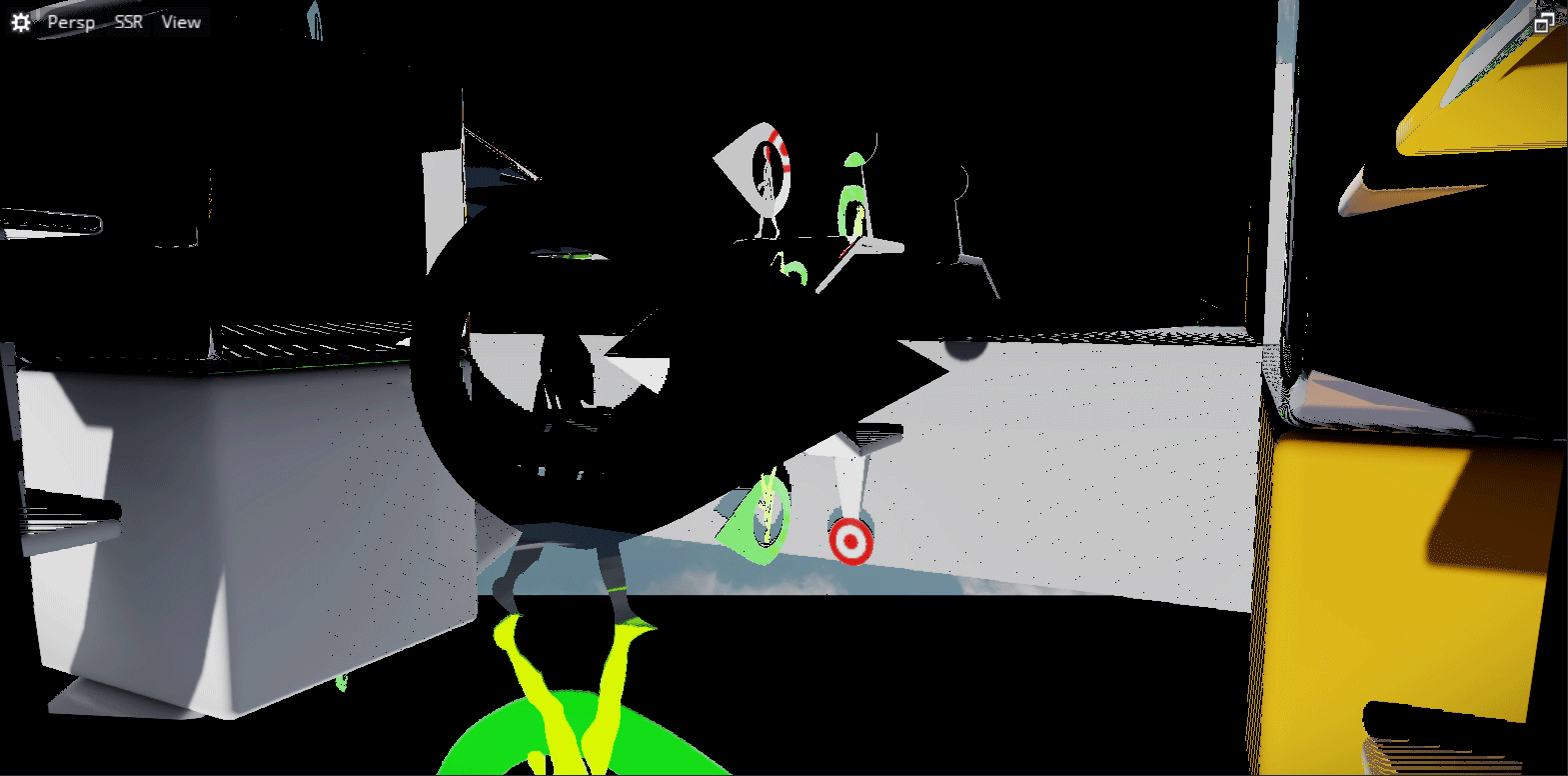This looked better. However it didn't address all of the tracing artifacts we were seeing. The results were still plagued with lots of small pixels whose traced rays failed. When investigating these failing cases I noticed that they would sometimes get stuck for no apparent reason in a cell along the way. It also occurred more frequently when rays travelled in the screen space axes (±1,0) or (0,±1). After drawing a bunch of ray diagrams on paper I realized that the cell intersection method proposed in GPU-Pro had a failing case! To ensure hiz cells are always crossed, the article offsets the intersection planes of a cell by a small offset. This is to ensure that the intersection point crosses the boundaries of the cell it's intersecting so that the trace continues to make progress.

While this works in most cases there is one scenario which results in a ray that will not cross over into the next hiz cell (see diagram bellow). When this happens the ray wastes the rest of it's allocated trace iterations intersecting the same cell without ever crossing it. To address this we changed the proposed method slightly. Instead of offsetting the bounding planes, we choose the appropriate offset to add depending on which plane was intersected (horizontal or vertical). This ensures that we will always cross a cell when tracing: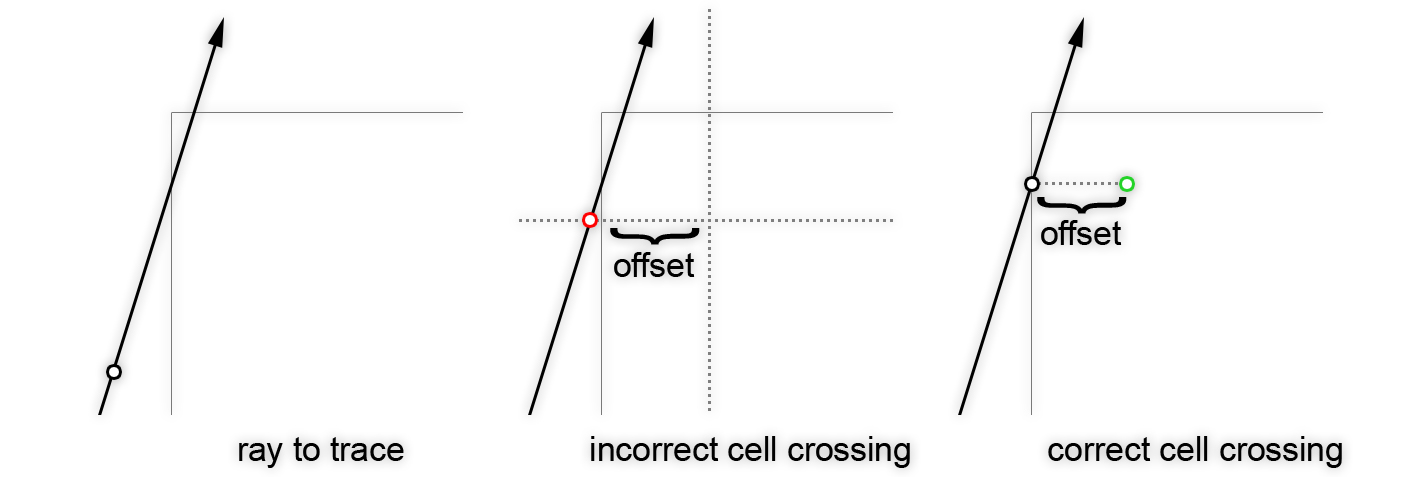``````float2 cell_size = 1.0 / cell_count;
float2 planes = cell_id/cell_count + cell_size * cross_step + cross_offset;
float2 solutions = (planes - pos.xy)/dir.xy;

float3 intersection_pos = pos + dir * min(solutions.x, solutions.y);
return intersection_pos;

float2 planes = cell_id/cell_count + cell_size * cross_step;
float2 solutions = (planes - pos)/dir.xy;

float3 intersection_pos = pos + dir * min(solutions.x, solutions.y);
intersection_pos.xy += (solutions.x < solutions.y) ? float2(cross_offset.x, 0.0) : float2(0.0, cross_offset.y);
return intersection_pos;``````
Incorrect VS correct cell crossing: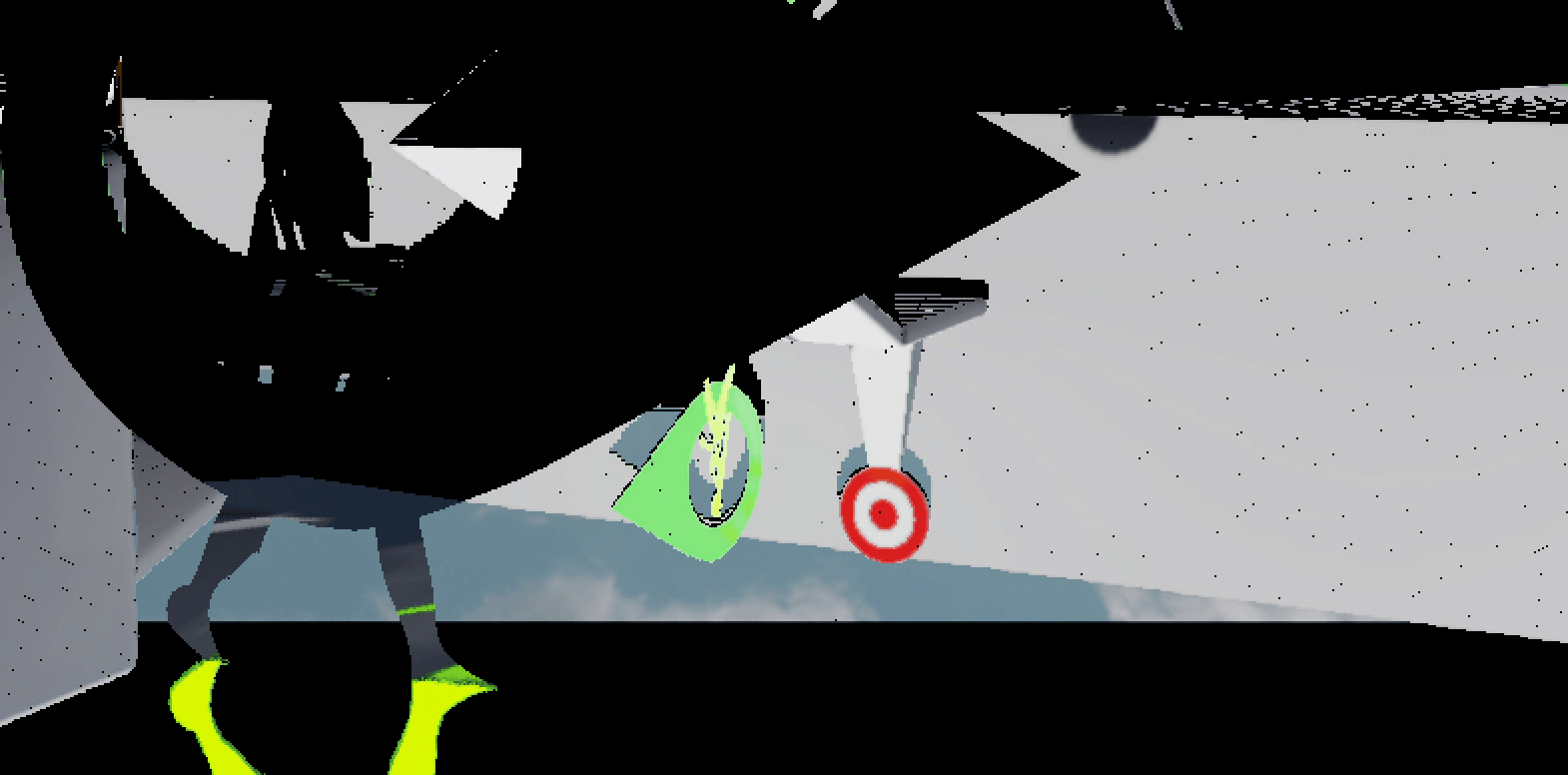Final Result: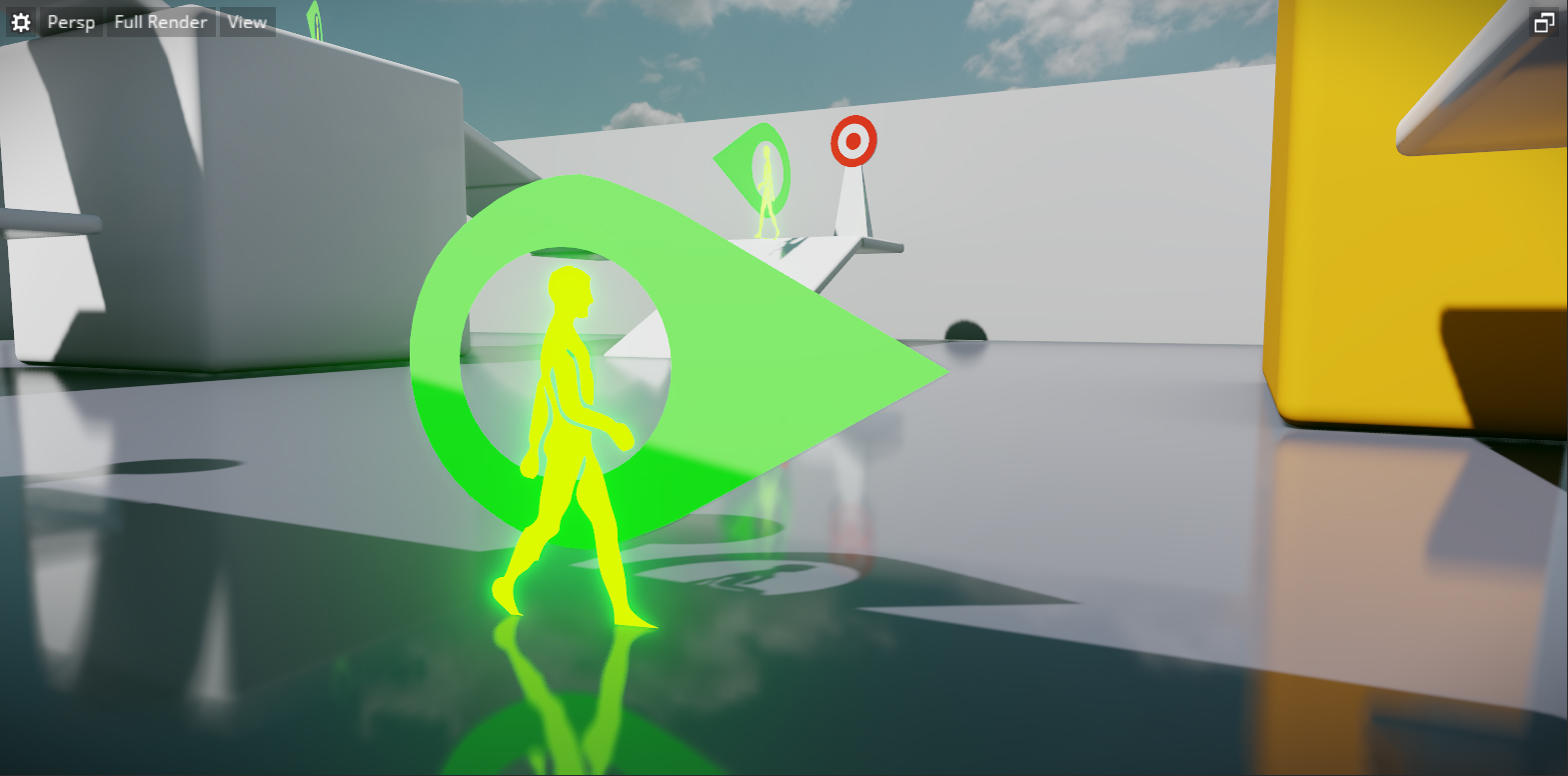## Ray Marching Towards the Camera

At the end of the GPU-Pro chapter there is a small mention that raymarching towards the camera with hiz tracing would require storing both the minimum and maximum depth value in the hiz structure (requiring to bump the format to a R32G32F format). However if you visualize the trace of a ray leaving the surface and travelling towards the camera (i.e. away from the depth buffer plane) then you can simply acount for that case and augment the algorithm described in GPU-Pro to navigate up and down the hierarchy until the ray finds the first hit with a hiz cell: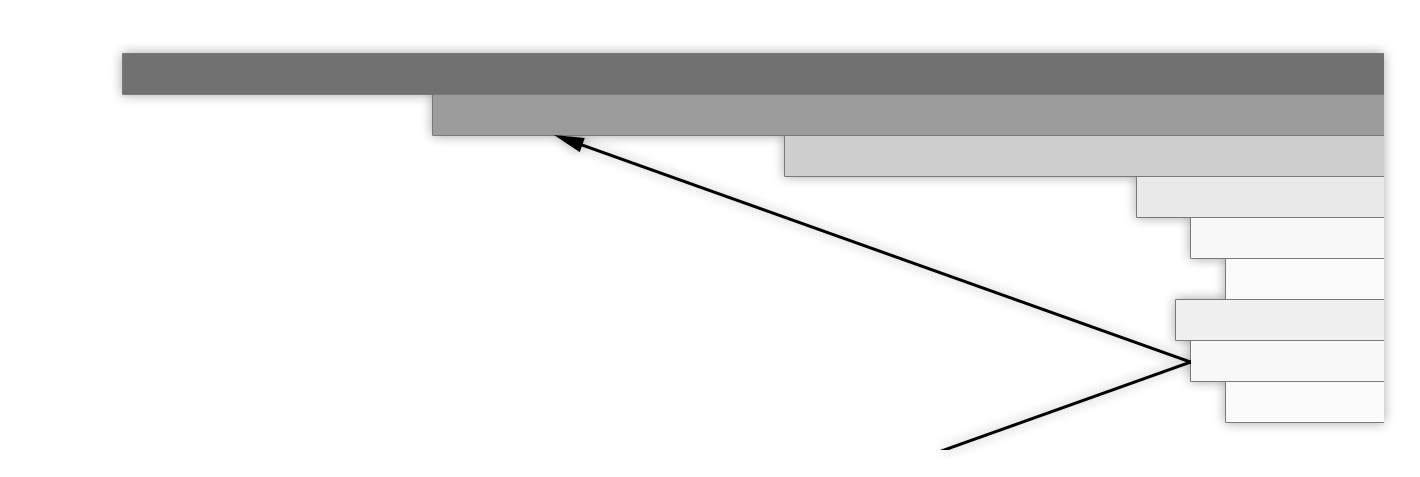``````if(v.z > 0) {
float min_minus_ray = min_z - ray.z;
tmp_ray = min_minus_ray > 0 ? ray + v_z*min_minus_ray : tmp_ray;
float2 new_cell_id = cell(tmp_ray.xy, current_cell_count);
if(crossed_cell_boundary(old_cell_id, new_cell_id)) {
tmp_ray = intersect_cell_boundary(ray, v, old_cell_id, current_cell_count, cross_step, cross_offset);
level = min(HIZ_MAX_LEVEL, level + 2.0f);
}
} else if(ray.z < min_z) {
tmp_ray = intersect_cell_boundary(ray, v, old_cell_id, current_cell_count, cross_step, cross_offset);
level = min(HIZ_MAX_LEVEL, level + 2.0f);
}``````

This has proven to be fairly solid and enabled us to trace a wider range of the screen space:

Another alteration that can be made to the hiz tracing algorithm is to add support for rays to travel behind surface. Of course to do this you must define a thickness to the surface of the hiz cells. So instead of tracing against extruded hiz cells you trace against "floating" hiz cells.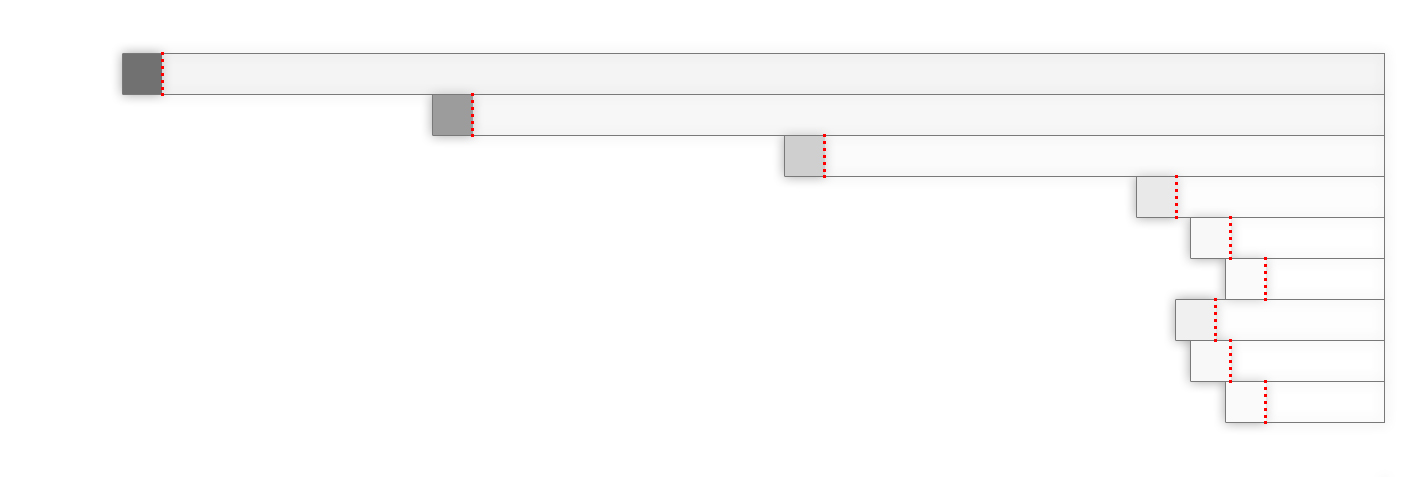With that in mind we can tighten the tracing algorithm so that it cannot end the trace unless it finds a collision with one of these floating cells:

``````if(level == HIZ_START_LEVEL && min_minus_ray > depth_threshold) {
tmp_ray = intersect_cell_boundary(ray, v, old_cell_id, current_cell_count, cross_step, cross_offset);
level = HIZ_START_LEVEL + 1;
}``````

Tracing behind surfaces disabled VS enabled: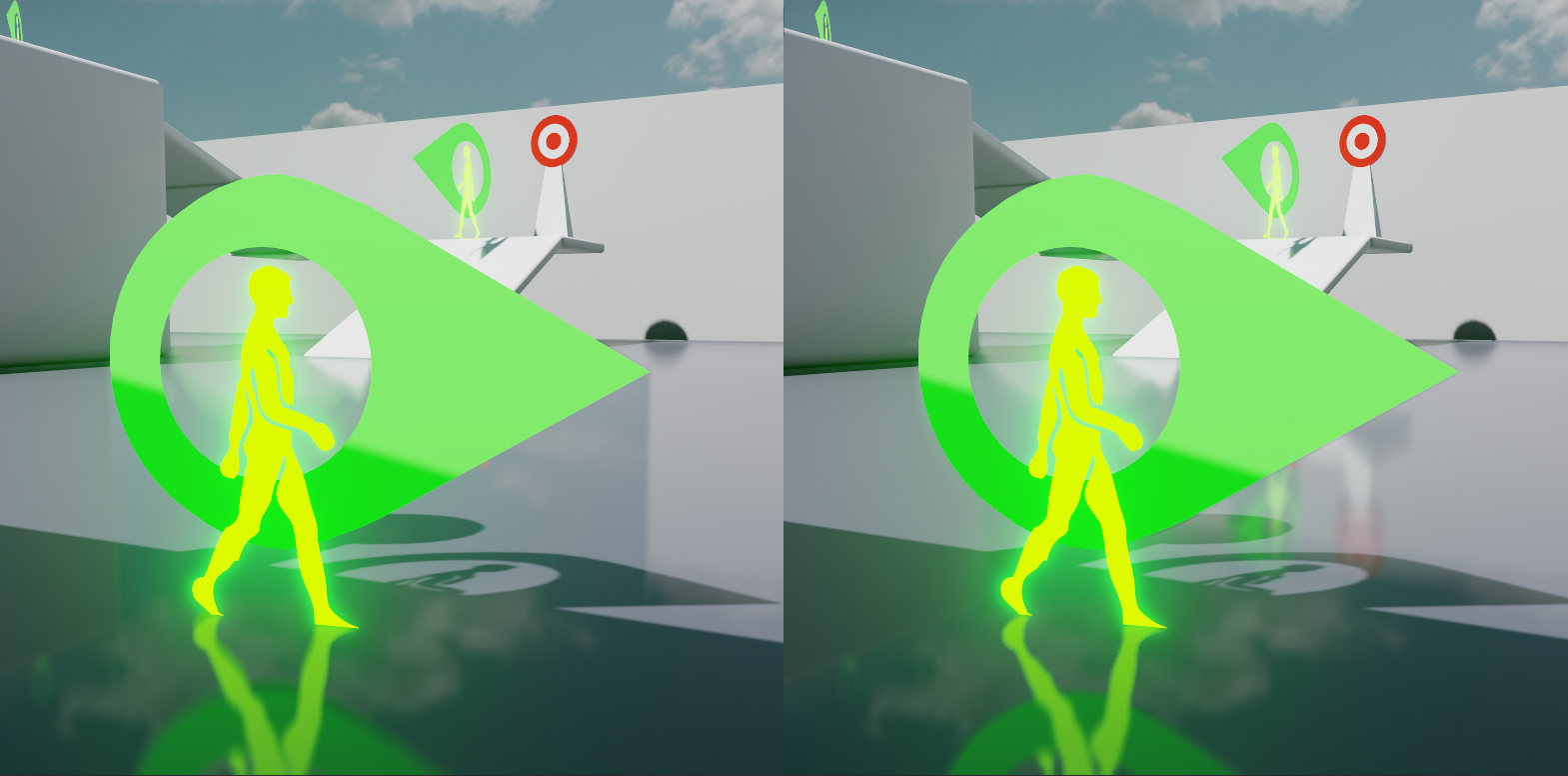Tracing behind surfaces disabled VS enabled: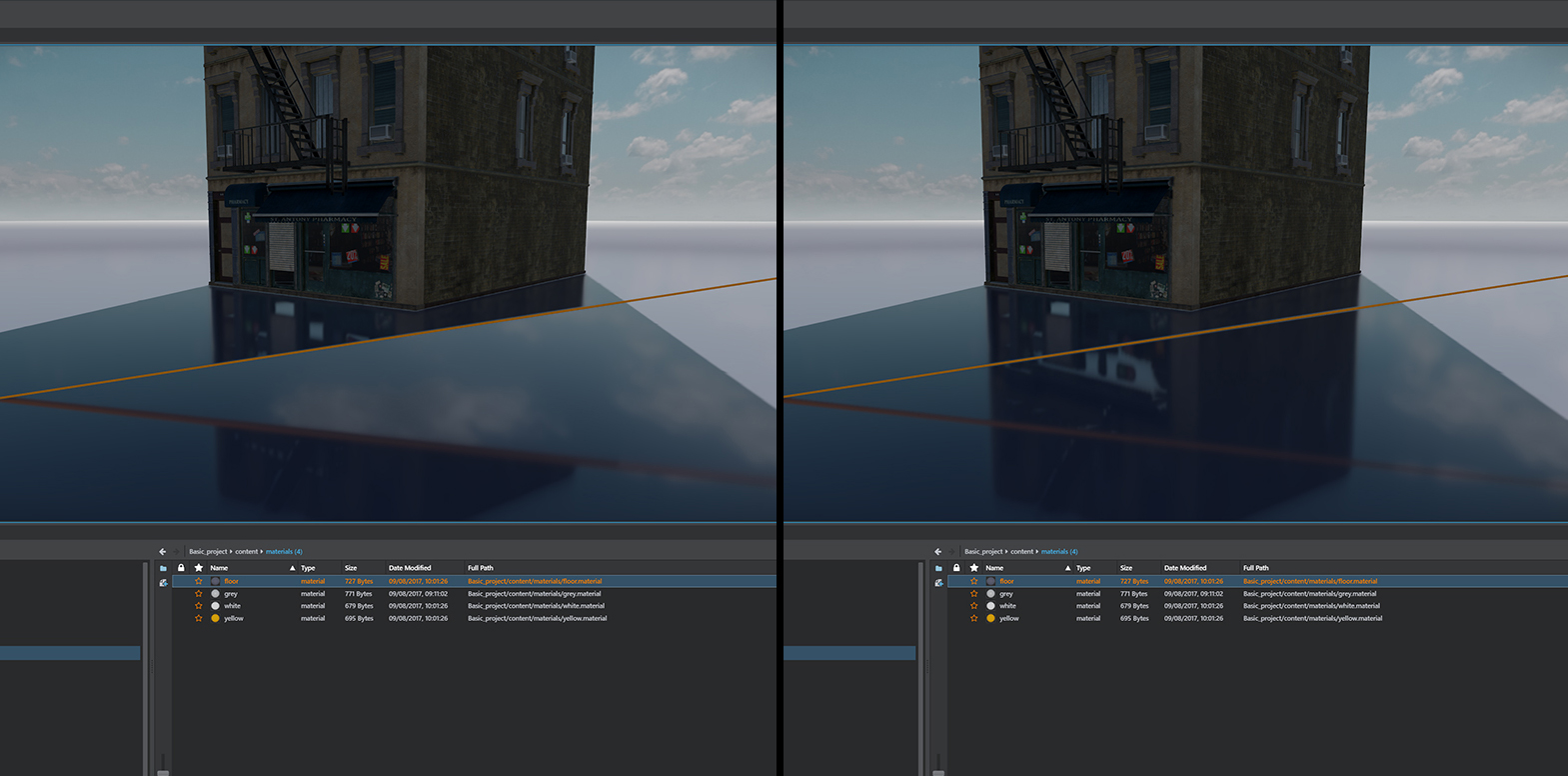Unfortunately this often means that the traced rays travelling behind a surface degenerate into a linear search and the cost can skyrocket for these traced pixels:

Number of iterations to complete the trace (black=0, red=64):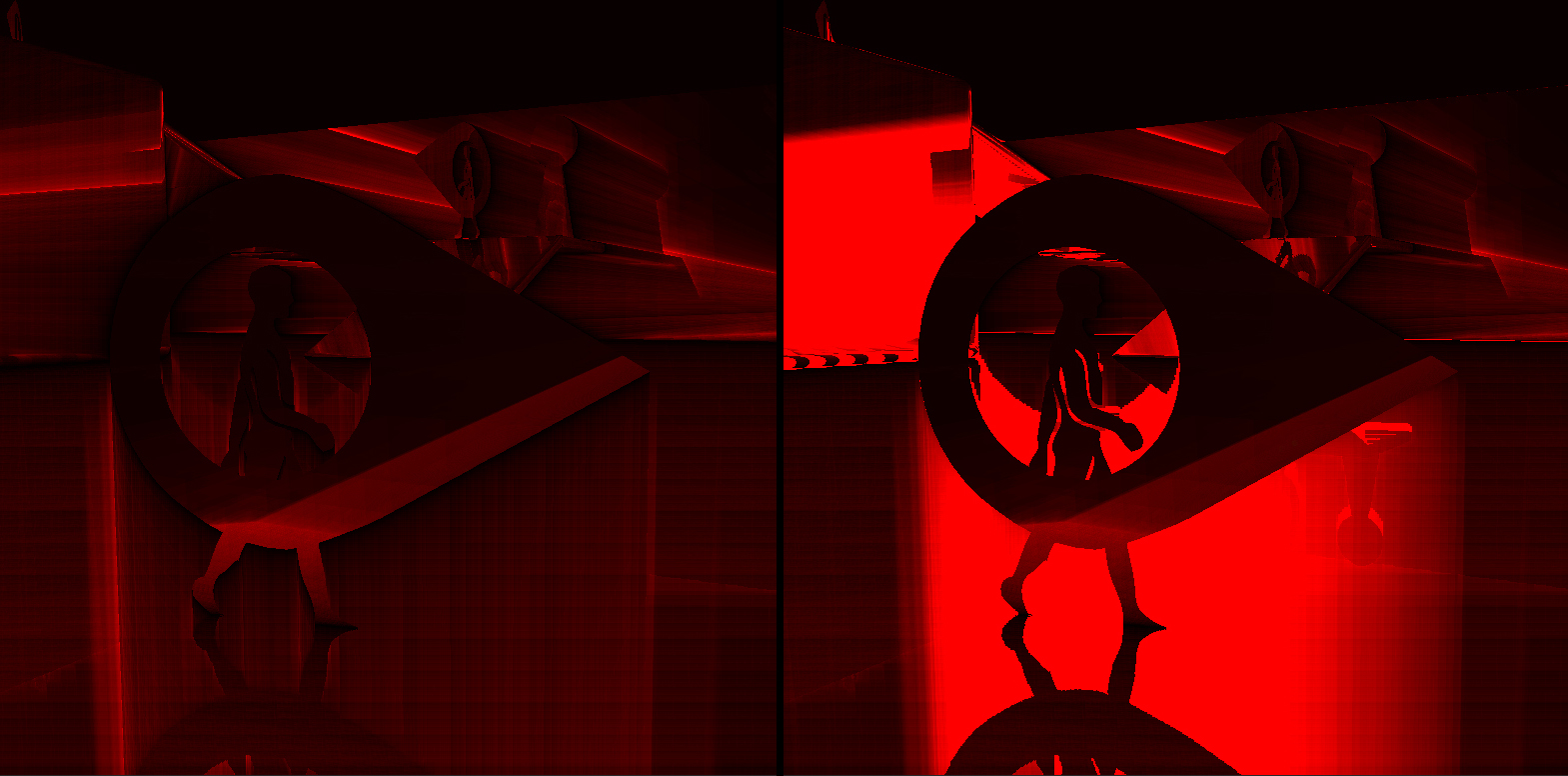For me the most difficult artifact to understand and deal with when implementing ssr is (by far) the implications of tracing a discreet depth buffer. Unless you can fully commit to the idea of tracing objects with infinite thicknesses, you will need to use some kind of depth threshold to mask a reflection if it's intersection with the geometry is not valid. If you do use a depth threshold then you can (will?) end up getting artifacts like these:

The problem as far as I understand it, is that rays can osciliate from passing and failing the depth threshold test. It is essentially an amplified alliasing problem caused by the finite resolution of the depth buffer: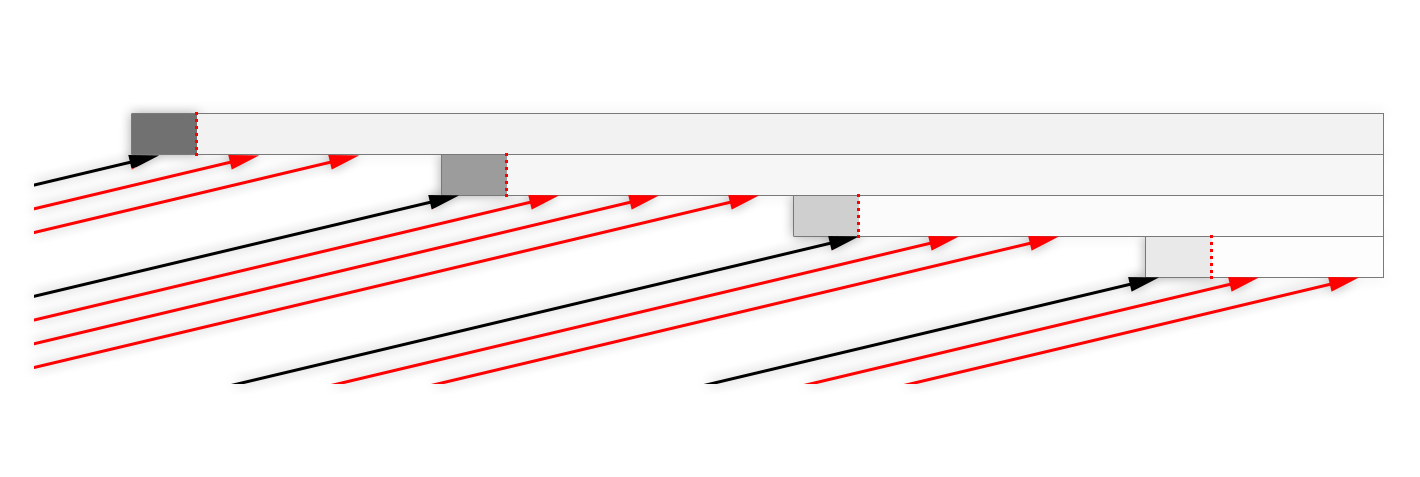I have experimented with adapting the depth threshold based on different properties of the intersection point (direction of reflected ray, angle of insidence at intersection, surface inclination at intersection) but I have never been able to find a silver bullet (or anything that resembles a bullet to be honest). Perhaps a good approach could be to interpolate the depth value of neighboring cells if the neighbors belong to the same geometry? I think that Mikkel Svendsen proposed a solution to this problem while presenting Low Complexity, High Fidelity: The Rendering of "INSIDE" but I have yet to wrap my head around the proposed solution and try it.

Finally it's worth pointing out that hiz tracing is a very "all or nothing" way to find an intersection point. Neighboring rays that exhaust their maximum number of allowed iterations to find an intersection can end up in very different screen spaces which can cause a noticeable discontinuity in the ssr buffer: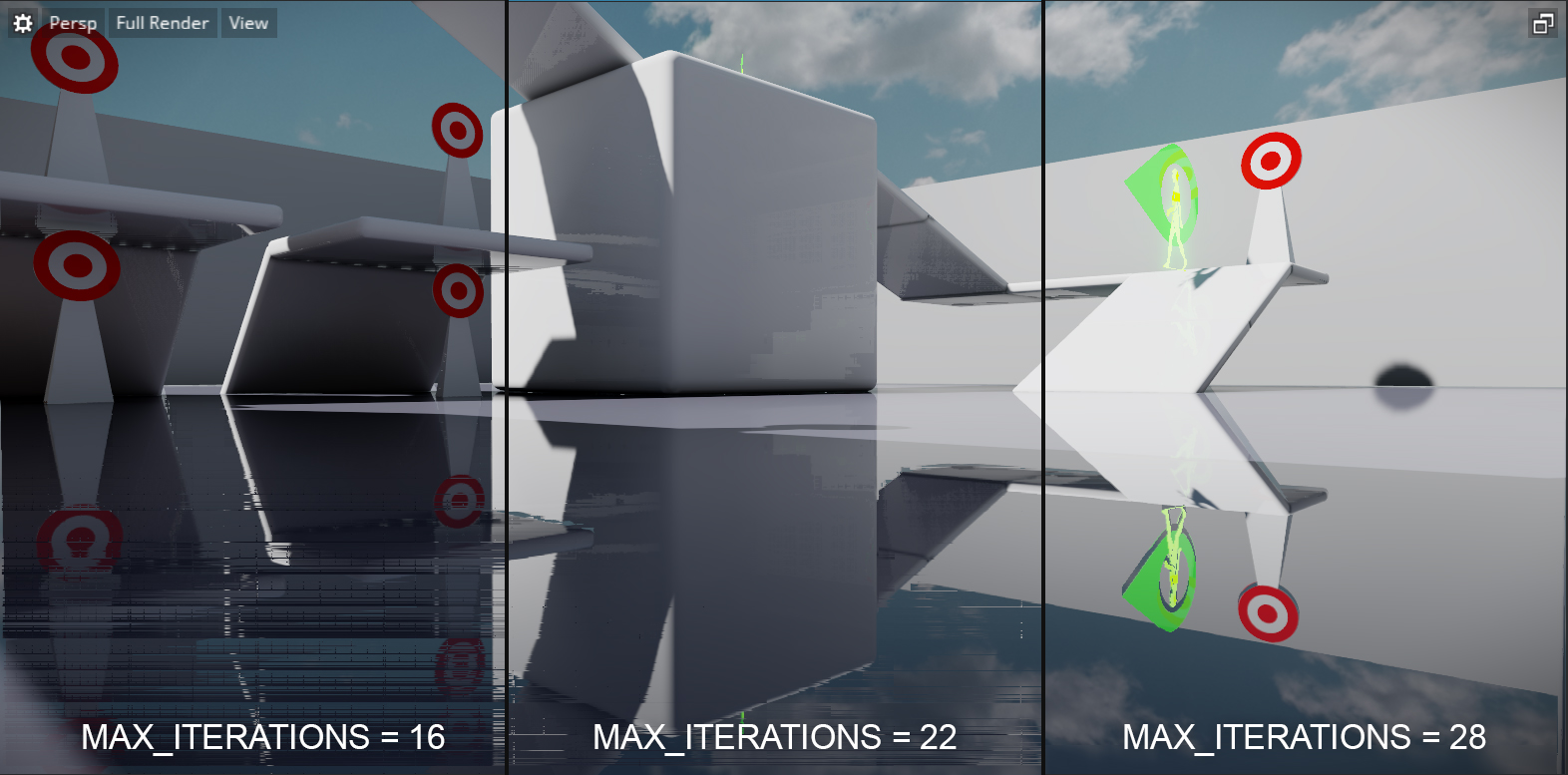This is something that can be very distracting and made much worst when dealing with a jittered depth buffer when combined with taa. This side-effect should be considered carefully when choosing a tracing solution for ssr.

## Code

``````float2 cell(float2 ray, float2 cell_count, uint camera) {
return floor(ray.xy * cell_count);
}

float2 cell_count(float level) {
return input_texture2_size / (level == 0.0 ? 1.0 : exp2(level));
}

float3 intersect_cell_boundary(float3 pos, float3 dir, float2 cell_id, float2 cell_count, float2 cross_step, float2 cross_offset, uint camera) {
float2 cell_size = 1.0 / cell_count;
float2 planes = cell_id/cell_count + cell_size * cross_step;

float2 solutions = (planes - pos)/dir.xy;
float3 intersection_pos = pos + dir * min(solutions.x, solutions.y);

intersection_pos.xy += (solutions.x < solutions.y) ? float2(cross_offset.x, 0.0) : float2(0.0, cross_offset.y);

return intersection_pos;
}

bool crossed_cell_boundary(float2 cell_id_one, float2 cell_id_two) {
return (int)cell_id_one.x != (int)cell_id_two.x || (int)cell_id_one.y != (int)cell_id_two.y;
}

float minimum_depth_plane(float2 ray, float level, float2 cell_count, uint camera) {
return input_texture2.Load(int3(vr_stereo_to_mono(ray.xy, camera) * cell_count, level)).r;
}

float3 hi_z_trace(float3 p, float3 v, in uint camera, out uint iterations) {
float level = HIZ_START_LEVEL;
float3 v_z = v/v.z;
float2 hi_z_size = cell_count(level);
float3 ray = p;

float2 cross_step = float2(v.x >= 0.0 ? 1.0 : -1.0, v.y >= 0.0 ? 1.0 : -1.0);
float2 cross_offset = cross_step * 0.00001;
cross_step = saturate(cross_step);

float2 ray_cell = cell(ray.xy, hi_z_size.xy, camera);
ray = intersect_cell_boundary(ray, v, ray_cell, hi_z_size, cross_step, cross_offset, camera);

iterations = 0;
while(level >= HIZ_STOP_LEVEL && iterations < MAX_ITERATIONS) {
// get the cell number of the current ray
float2 current_cell_count = cell_count(level);
float2 old_cell_id = cell(ray.xy, current_cell_count, camera);

// get the minimum depth plane in which the current ray resides
float min_z = minimum_depth_plane(ray.xy, level, current_cell_count, camera);

// intersect only if ray depth is below the minimum depth plane
float3 tmp_ray = ray;
if(v.z > 0) {
float min_minus_ray = min_z - ray.z;
tmp_ray = min_minus_ray > 0 ? ray + v_z*min_minus_ray : tmp_ray;
float2 new_cell_id = cell(tmp_ray.xy, current_cell_count, camera);
if(crossed_cell_boundary(old_cell_id, new_cell_id)) {
tmp_ray = intersect_cell_boundary(ray, v, old_cell_id, current_cell_count, cross_step, cross_offset, camera);
level = min(HIZ_MAX_LEVEL, level + 2.0f);
}else{
if(level == 1 && abs(min_minus_ray) > 0.0001) {
tmp_ray = intersect_cell_boundary(ray, v, old_cell_id, current_cell_count, cross_step, cross_offset, camera);
level = 2;
}
}
} else if(ray.z < min_z) {
tmp_ray = intersect_cell_boundary(ray, v, old_cell_id, current_cell_count, cross_step, cross_offset, camera);
level = min(HIZ_MAX_LEVEL, level + 2.0f);
}

ray.xyz = tmp_ray.xyz;
--level;

++iterations;
}
return ray;
}``````
Note: Original post made on the: Autodesk Stingray Blog